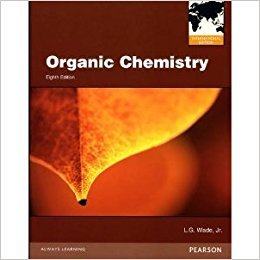×
×

# Solutions for Chapter Chapter 23: Carbohydrates and Nucleic Acids## Full solutions for Organic Chemistry | 8th Edition

ISBN: 9780321811394Solutions for Chapter Chapter 23: Carbohydrates and Nucleic Acids

Solutions for Chapter Chapter 23
4 5 0 259 Reviews
27
3
##### ISBN: 9780321811394

This textbook survival guide was created for the textbook: Organic Chemistry, edition: 8. Organic Chemistry was written by and is associated to the ISBN: 9780321811394. Chapter Chapter 23: Carbohydrates and Nucleic Acids includes 73 full step-by-step solutions. Since 73 problems in chapter Chapter 23: Carbohydrates and Nucleic Acids have been answered, more than 19900 students have viewed full step-by-step solutions from this chapter. This expansive textbook survival guide covers the following chapters and their solutions.

Key Chemistry Terms and definitions covered in this textbook
• acid

A substance that is able to donate a H+ ion (a proton) and, hence, increases the concentration of H+1aq2 when it dissolves in water. (Section 4.3)

• base ionization constant (Kb).

The equilibrium constant for the base ionization. (15.6)

• catalyst

A substance that changes the speed of a chemical reaction without itself undergoing a permanent chemical change in the process. (Section 14.7)

• Chain termination

A step in a chain reaction that involves destruction of reactive intermediates

• Coulomb’s law.

The potential energy between two ions is directly proportional to the product of their charges and inversely proportional to the distance between them. (9.3)

• dynamic equilibrium

A state of balance in which opposing processes occur at the same rate. (Section 11.5)

• fossil fuels

Coal, oil, and natural gas, which are presently our major sources of energy. (Section 5.8)

• half-reaction

An equation for either an oxidation or a reduction that explicitly shows the electrons involved, for example, Zn2 + 1aq2 + 2 e- ¡ Zn1s2. (Section 20.2)

• Homotopic groups

Atoms or groups on an atom that give an achiral molecule when one of the groups is replaced by another group. The hydrogens of the CH2 group of propane, for example, are homotopic. Replacing either one of them with deuterium gives 2-deuteropropane, which is achiral. Homotopic groups have identical chemical shifts under all conditions

• ion–dipole force

The force that exists between an ion and a neutral polar molecule that possesses a permanent dipole moment. (Section 11.2)

• metal complex

An assembly of a metal ion and the Lewis bases bonded to it. (Section 23.2)

• molecular equation

A chemical equation in which the formula for each substance is written without regard for whether it is an electrolyte or a nonelectrolyte. (Section 4.2)

• optically pure

A solution containing just one enantiomer, but not its mirror image.

• polyether

A compound containing several ether groups.

• primary structure

The sequence of amino acids along a protein chain. (Section 24.7)

• quantitative analysis

The determination of the amount of a given substance that is present in a sample. (Section 17.7)

• Reductive elimination

Elimination of two substituents at a metal center, causing the oxidation state of the metal to decrease by two.

• saturated

A compound that contains no p bonds.

• symmetrical ether

An ether (R!O!R) where both R groups are identical.

• Terpene

A compound whose carbon skeleton can be divided into two or more units identical with the carbon skeleton of isoprene

×